# Rounding of Money Amounts

Deutsch

Many numerical calculations deal with amounts of money. It is always nice if these calculations are somewhat correct or if we can at least rest assured that we do not loose money due to such inaccuracies. Off course there are calculations that may legitimately deal with approximations, for example when calculating profits as percentages of the investment (return on investment). For this kind of calculations floating point numbers (double, Float or something like that) come in handy, but off course dealing with the numeric subtleties can be quite a challenge and rounding errors can grow huge if not dealt with properly.

It is often necessary to do calculations with exact amounts. It is quite easy to write something like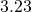as floating point number, but unfortunately these are internally expressed in binary format (base 2), so the fraction with a power of 10 in the denominator needs to be expressed as a fraction with a power of two in the denominator. We can just give it a try and divide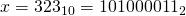by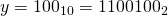, doing the math in base 2 arithmetic. We get something like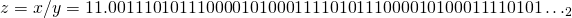, more preciselyor as fraction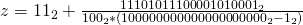or in base 10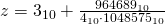.

It can be seen that such a harmless number with only two digits after the decimal point ends up having an infinite number of digits after the decimal point. Our usual Double and Float types are usually limited to 64 Bits, so some digits have to be discarded, causing a rounding error. Fortunately this usually works well and the stored number is still shown as. But working a little bit with these floating point numbers sooner or later results like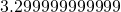orwill appear instead of, even when doing only addition and subtraction. When doing larger sums it might even end up as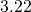or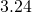. For many applications that is unacceptable.

A simple and often useful solution is to use integral numbers and storing the amounts in cents instead of dollars. Then the rounding problem of pure additions and subtractions is under control and for other operations it might at least become easier to deal with the issue. A common mistake that absolutely needs to be avoided is mixing amountInDollar and amountInCent. In Scala it would be a good idea to use different types to make this distinction, so that such errors can be avoided at compile time. In any case it is very important to avoid such numeric types as int of Java that have a weird and hardly controllable overflow behavior where adding positive numbers can end up negative. How absurd, modular arithmetic is for sure not what we want for our money, it is a little bit too socialistic. 😉 Estimations about the upper bound of money amounts of persons and companies and other organizations are problematic, because there can be inflation and there can be some accumulation of money in somebody’s accounts… Even though databases tend to force us to such assumption, but we can make them really huge. So some languages might end up using something like BigInteger or BigNum or BigInt. Unfortunately Java shows one of its insufficiencies here, which makes quite ugly to use for financial applications, because calculations like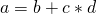for BigInteger appear like this: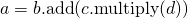. The disadvantage is that the formula cannot be seen at one glance, which leads to errors. In principal this problem can be solved using a preprocessor for Java. Maybe a library doing some kind of RPN-notation would possible, writing code like this:

Calculation calc = new Calculation();
calc.push(b)
calc.push(c)
calc.push(d)
calc.multiply()
a = calc.top()


Those who still know old HP calculators (like HP 25 and HP 67 😉 in the good old days) or Forth might like this, but for most of us this is not really cutting it.

Common and useful is actually the usage of some decimal fixed point type. In Java this is BigDecimal, in Ruby it is LongDecimal.
And example in Ruby:

> sudo gem install long-decimal
Successfully installed long-decimal-1.00.01
1 gem installed
....
> irb
irb(main):001:0> require "long-decimal"
=> true
irb(main):002:0> x = LongDecimal("3.23")
=> LongDecimal(323, 2)
irb(main):003:0> y = LongDecimal("7.68")
=> LongDecimal(768, 2)
irb(main):004:0> z = LongDecimal("3.9291")
=> LongDecimal(39291, 4)
irb(main):005:0> x+y
=> LongDecimal(1091, 2)
irb(main):006:0> (x+y).to_s
=> "10.91"
irb(main):007:0> x+y*z
=> LongDecimal(33405488, 6)
irb(main):008:0> (x+y*z).to_s
=> "33.405488"
irb(main):009:0>


It is interesting to see that the number of digits remains the same under addition and subtraction if both sides have the same number of digits. But the longer number of digits wins otherwise. During multiplication, division and off course during more complex operations many decimal places can become necessary. It becomes important to do rounding and to do it right and in a controlled way. LongDecimal supports the following rounding modes:

ROUND_UP
Round away from 0.
ROUND_DOWN
Round towards 0.
ROUND_CEILING
Round to more positive, less negative numbers.
ROUND_FLOOR
Round to more negative, less positive numbers.
ROUND_HALF_UP
Round the middle and from above up (away from 0), everything below down (towards 0).
ROUND_HALF_DOWN
Round the middle and from above down (towards 0), everything below up (away from 0).
ROUND_HALF_CEILING
Round from the middle onward towards infinity, otherwise towards negative infinity.
ROUND_HALF_FLOOR
Round up to and including the middle towards negative infinity, otherwise towards infinity.
ROUND_HALF_EVEN
Round the middle in such a way that the last digit becomes even.
ROUND_HALF_ODD
Round the middle in such a way that the last digit becomes odd (will be added to long-decimal in next release).
ROUND_UNNECESSARY
Do not round, just discard trailing 0. If that does not work, raise an exception.

Which of these to use should be decided with domain knowledge in mind. The above example could be continued as follows:

irb(main):035:0> t=(x+y*z)
=> LongDecimal(33405488, 6)
irb(main):036:0>
irb(main):037:0* t.round_to_scale(2, LongDecimal::ROUND_UP).to_s
=> "33.41"
irb(main):038:0> t.round_to_scale(2, LongDecimal::ROUND_DOWN).to_s
=> "33.40"
irb(main):039:0> t.round_to_scale(2, LongDecimal::ROUND_CEILING).to_s
=> "33.41"
irb(main):040:0> t.round_to_scale(2, LongDecimal::ROUND_FLOOR).to_s
=> "33.40"
irb(main):041:0> t.round_to_scale(2, LongDecimal::ROUND_HALF_UP).to_s
=> "33.41"
irb(main):042:0> t.round_to_scale(2, LongDecimal::ROUND_HALF_DOWN).to_s
=> "33.41"
irb(main):043:0> t.round_to_scale(2, LongDecimal::ROUND_HALF_CEILING).to_s
=> "33.41"
irb(main):044:0> t.round_to_scale(2, LongDecimal::ROUND_HALF_FLOOR).to_s
=> "33.41"
irb(main):045:0> t.round_to_scale(2, LongDecimal::ROUND_HALF_EVEN).to_s
=> "33.41"
irb(main):046:0> t.round_to_scale(2, LongDecimal::ROUND_UNNECESSARY).to_s
ArgumentError: mode ROUND_UNNECESSARY not applicable, remainder 5488 is not zero
from /usr/local/lib/ruby/gems/1.9.1/gems/long-decimal-1.00.01/lib/long-decimal.rb:507:in round_to_scale_helper'
from /usr/local/lib/ruby/gems/1.9.1/gems/long-decimal-1.00.01/lib/long-decimal.rb:858:in round_to_scale'
from (irb):46
from /usr/local/bin/irb:12:in '
irb(main):047:0>


A specialty is that some countries do not use the coins with one of the smallest unit, like 1 cent in the US. In Switzerland the smallest common coin is 5 Rp (5 cent=0.05 CHF). It might be possible to make the bank do a transfer for amounts not ending in 0 or 5, but usually invoices apply this kind of rounding and avoid such exact amounts that could not possibly be paid in cash. This can be dealt with by multiplying the amount by 20, rounding it to 0 digits after the decimal point and divide it by 20 and round the result to 2 digits after the point. In Ruby there is a better way using an advanced feature of LongDecimal called remainder rounding (using the method round_to_allowed_remainders(…) ). Assuming we want to round a number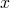with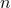decimal places in such a way that,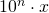belongs to one of the residue classesor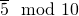. In this case we are just talking about the last digit, but the mechanism has been implemented in a more general way allowing any set of allowed residues and even a base other than 10. If 0 is not in the set of allowed residues, it may be unclear how 0 should be rounded and this needs to be actually defined with a parameter. For common practical uses the last digit of 0 is allowed, so things work out of the box:


irb(main):003:0> t.round_to_allowed_remainders(2, [0, 5], 10, LongDecimal::ROUND_UP).to_s
=> "33.45"
irb(main):005:0> t.round_to_allowed_remainders(2, [0, 5], 10, LongDecimal::ROUND_DOWN).to_s
=> "33.40"
irb(main):006:0> t.round_to_allowed_remainders(2, [0, 5], 10, LongDecimal::ROUND_CEILING).to_s
=> "33.45"
irb(main):007:0> t.round_to_allowed_remainders(2, [0, 5], 10, LongDecimal::ROUND_FLOOR).to_s
=> "33.40"
irb(main):008:0> t.round_to_allowed_remainders(2, [0, 5], 10, LongDecimal::ROUND_HALF_UP).to_s
=> "33.40"
irb(main):009:0> t.round_to_allowed_remainders(2, [0, 5], 10, LongDecimal::ROUND_HALF_DOWN).to_s
=> "33.40"
irb(main):010:0> t.round_to_allowed_remainders(2, [0, 5], 10, LongDecimal::ROUND_HALF_CEILING).to_s
=> "33.40"
irb(main):011:0> t.round_to_allowed_remainders(2, [0, 5], 10, LongDecimal::ROUND_HALF_FLOOR).to_s
=> "33.40"
`

In an finance application the rounding methods should probably be defined for each currency, possible using one formula and some currency specific parameters.

LongDecimal can do even more than that. It is possible to calculate logarithms, exponential functions, square root, cube root all to a desired number of decimal places. The algorithms have been tuned for speed without sacrificing precision.